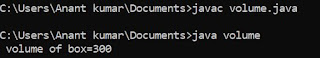## Thursday, 12 July 2018

### Program to calculate sum of two matrix of order m * n in C using for loop statement

/* addition of matrix */
#include<stdio.h>
void main ()
{
int  ,  ,  ;
int  r1 , r2 , c1 , c2 ;
int i , j ;
clrscr () ;
printf ("\n Enter the no of rows for first element:") ;
scanf ("%d", &r1) ;
printf ("\n Enter the no of column for first element:") ;
scanf ("%d", &c1) ;
printf ("\n Enter the no of rows for second element:") ;
scanf ("%d", &r2) ;
printf ("\n Enter the no of column for second element:") ;
scanf ("%d", &c2) ;
if ( ( r1!=r2) && (c1!=c2) )
{
printf ("\n addition not possible:") ;
getch () ;
exit () ;
}
for ( i = 0 ; i < r1 ; i++ )                   /* Input of first matrix */
{
for ( j = 0 ; j < c1 ; j++ )
{
printf ("\n Enter a number:") ;
scanf ("%d", & a {i}{j}) ;
}
}
for ( i = 0 ; i < r2 ; i++ )                    /* Input of second matrix */
{
for ( j = 0 ; j < c2 ; j++ )
{
printf ("\n Enter a number:") ;
scanf ("%d", & b {i}{j}) ;
}
}
for ( i = 0 ; i < r1 ; i++ )             /* print first matrix */
{
for ( j = 0 ; j < c1 ; j++ )
printf ("%3 d", a {i}{j}) ;
printf ("\n") ;
}
for ( i = 0 ; i < r2 ; i++ )              /* print second matrix */
{
for ( j = 0 ; j < c2 ; j++ )
printf ("%3 d", b{i}{j}) ;
printf ("\n") ;
}

for ( i = o ; i < r1 ; i++ )                   /* calculation of matrix */
{
for ( j = 0 ; j < c1 ; j++ )
{
c {i}{j} = a {i}{j} + b {i}{j} ;
}
}
for ( i = 0 ; i < r1; i++ )
{
for ( j = 0 ; j < c1: j++ )
printf ("%3 d", c {i}{j}) ;

printf ("\n") ;
}
getch () ;
}

### Topics used :

In C programming array is very important topic as we discuss earlier there is two types of array in c you may read at https://www.programmingponds.com/2018/07/array-in-c.html and at https://www.programmingponds.com/2018/07/c-array-types.html .

### How to add two matrix :

As you seen in the program first of all we have to declare the variable . This is program of m * n matrix but we have initialized the value of m and n otherwise there is waste of huge memory and the value of m and n must be initialized according to the use of user .

As we know that for addition of matrix possible there must be number of rows of first matrix should be equal to the number of rows of second matrix and the number of column of first matrix is also equal to the number of column of second matrix for this we have to apply a conditional statement .